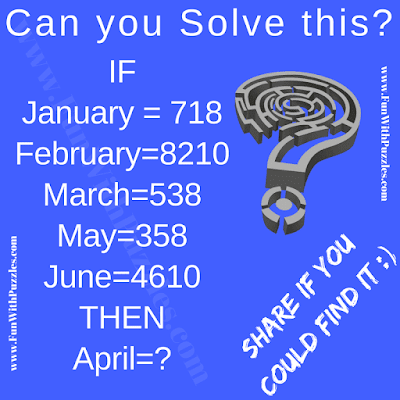Can the Months equates to numbers? Yes, Months can equates to the numbers. In this logic picture for teens puzzle, there are equations given in the puzzle picture. Left side of these equations contains name of the month and right side of the equation contains the numbers. These Month names and numbers relates to each other. Can you find this relationship and then find the value of April?Can you solve this logic picture puzzle?

Answer of this "Logic Picture for Teens", can be viewed by clicking on button. Please do give your best try before looking at the answer.